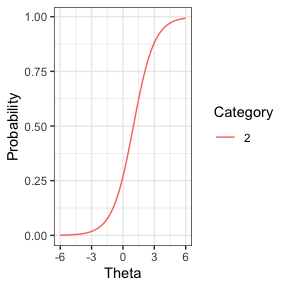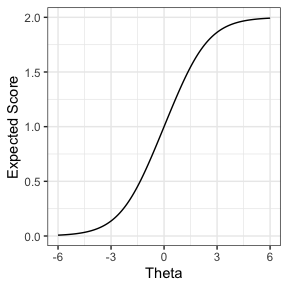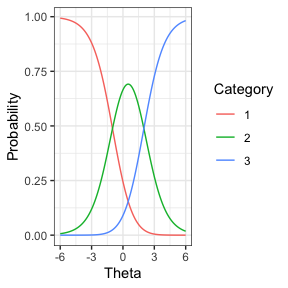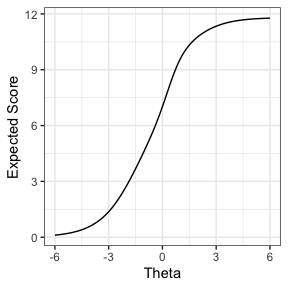# Introduction

Given a set of item parameters and a set of abilities, it is possible to simulate a set of item responses.

Users can also impose a factor structure that maps items to specific dimensions, and impose various missing data structures.

Users can also permute the discrimination of items to impose misfit on the simulated data.

# Example

## Simple case: unidimensional structure with Rasch-like items

The item parameters for a simple item are represented as:

library(conquestr)
myItem <- matrix(c(0, 0, 0, 0, 1, 1, 0, 1), ncol = 4, byrow = TRUE)
colnames(myItem) <- c("k", "d", "t", "a")
print(myItem)
#>      k d t a
#> [1,] 0 0 0 0
#> [2,] 1 1 0 1
plotModelCCC(myItem)where k is the category score, d is the delta dot ($$\dot{\delta}$$), t is the tau, and a is the discrimination parameter. By convention, the first row of the item matrix is zeros.

items are stored in lists, and a list of items can be visualised as the test characteristic curve which illustrates the expected raw score give an ability, theta ($$\Theta$$).

myItems <- list(myItem, myItem)
myItems[][2, 2] <- -1 # make the second item delta equal to -1
print(plotModelExp(myItems))In the unidminsaional case, abilities are a simple vector of abilities (for example, EAPs):

myAbils <- rnorm(100)

Given fixed item parameters and abilities, it I possible then to simulate item responses for each case. By default, there is no missing data and item response fit the item response model implied by the item parameters. The first 10 responses are shown, along with the person ability (column 3):

myResponses <- genResponses(myAbils, myItems)
print(cbind(myResponses[1:10, 1:2], myAbils[1:10]))
#>       [,1] [,2]       [,3]
#>  [1,]    0    1  0.7965057
#>  [2,]    0    0 -0.8527630
#>  [3,]    1    1  0.8427809
#>  [4,]    0    0 -0.7379118
#>  [5,]    0    0 -0.4384575
#>  [6,]    0    1 -1.5821600
#>  [7,]    1    1  1.1906216
#>  [8,]    1    1  0.2683473
#>  [9,]    0    1 -0.2535734
#> [10,]    1    1  0.5555316

## More complex case: multidimensional structure with a mixture of item types

A polytomous item can be described by including an item matrix with more than two rows. An item with its own discrimination parameter can be described by including the value in the a column. An item with non-integer scoring can be described by varying the value in the k column. Note that for identification purposes, the values in the t column should sum to zero, though this is not enforced to allow for sophisticated designs.

myItemPoly <- matrix(
c(
0, 0.0,  0.0, 0,
1, 0.5, -1.5, 1,
2, 0.5,  1.5, 1
),
ncol = 4, byrow = TRUE
)

myItem2Pl <- matrix(
c(
0,  0,  0.00, 0.00,
1, -2, -1.25, 0.85,
2, -2, -0.25, 0.85,
3, -2,  0.30, 0.85,
4, -2,  1.20, 0.85
),
ncol = 4, byrow = TRUE
)

myItemNonIntScore <- matrix(
c(
0,   0,  0.00, 0,
1.2, 0, -0.35, 1,
1.8, 0,  0.15, 1,
3.8, 0,  0.20, 1
),
ncol = 4, byrow = TRUE
)

myItems <- append(myItems, list(myItemPoly, myItem2Pl, myItemNonIntScore))
plotModelCCC(myItemPoly)plotModelExp(myItems)The multidimensional structure of the model is defined by allocating items to dimensions by constructing a B-matrix (sometimes called a scoring matrix – see Wilson, M., Zheng, X., & McGuire, L. (2012). Formulating latent growth using an explanatory item response model approach. Journal of Applied Measurement, 13(1), 1–22.). The B matrix maps items (rows) to dimensions (columns). Note that this is a simplified B matrix, in that it is strictly binary – any aspect of increasing coefficients (for example over time) should be captured in the scoring column, k, within the item matrix.

Note that now that the model is multidimensional, the abilities provided should also be a matrix, rather than a vector: cases (rows) by dimensions (columns). Any covariance structure amongst the dimensions is encoded within the matrix of abilities.

Note that one of the items does not use integer scoring. If the software to analyse the data assumes integer scoring, then secondary processing is required to yield traditional categorical data, and the scoring assumptions should be otherwise input into the software.

myBMatrix <- matrix(
c(
1, 0,
1, 0,
0, 1,
0, 1,
0, 1
),
byrow = TRUE,
ncol = 2
)

myAbils2 <- rnorm(length(myAbils))
myAbilsMat <- cbind(myAbils, myAbils2) # assumes expected correlation = 0

myResponses <- genResponses(myAbilsMat, myItems, BMatrix = myBMatrix)
print(cbind(myResponses[1:10, 1:5], myAbilsMat[1:10, 1:2]))
#>                      myAbils   myAbils2
#>  [1,] 0 1 2 4 3.8  0.7965057  1.3364925
#>  [2,] 0 1 0 3 1.2 -0.8527630 -1.2347370
#>  [3,] 1 1 1 4 3.8  0.8427809  0.6101917
#>  [4,] 0 1 1 4 3.8 -0.7379118  2.2117249
#>  [5,] 0 1 1 1 1.2 -0.4384575 -0.7333140
#>  [6,] 0 1 2 3 3.8 -1.5821600  0.2933033
#>  [7,] 0 0 1 4 3.8  1.1906216  0.8467243
#>  [8,] 0 0 1 3 0.0  0.2683473 -1.3508211
#>  [9,] 0 0 1 4 3.8 -0.2535734  0.1860145
#> [10,] 0 1 1 4 0.0  0.5555316  0.1555404